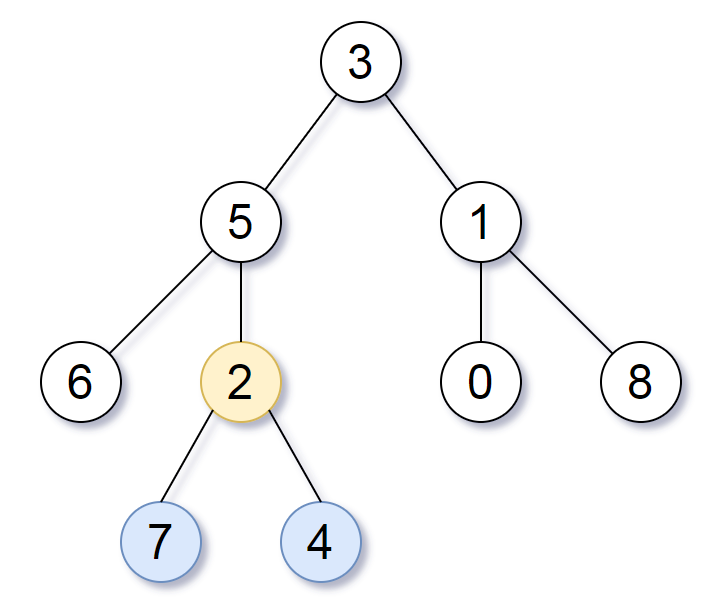## Algorithm

Problem Name: 865. Smallest Subtree with all the Deepest Nodes

Given the `root` of a binary tree, the depth of each node is the shortest distance to the root.

Return the smallest subtree such that it contains all the deepest nodes in the original tree.

A node is called the deepest if it has the largest depth possible among any node in the entire tree.

The subtree of a node is a tree consisting of that node, plus the set of all descendants of that node.

Example 1:```Input: root = [3,5,1,6,2,0,8,null,null,7,4]
Output: [2,7,4]
Explanation: We return the node with value 2, colored in yellow in the diagram.
The nodes coloured in blue are the deepest nodes of the tree.
Notice that nodes 5, 3 and 2 contain the deepest nodes in the tree but node 2 is the smallest subtree among them, so we return it.
```

Example 2:

```Input: root = 
Output: 
Explanation: The root is the deepest node in the tree.
```

Example 3:

```Input: root = [0,1,3,null,2]
Output: 
Explanation: The deepest node in the tree is 2, the valid subtrees are the subtrees of nodes 2, 1 and 0 but the subtree of node 2 is the smallest.
```

Constraints:

• The number of nodes in the tree will be in the range `[1, 500]`.
• `0 <= Node.val <= 500`

## Code Examples

### #1 Code Example with C Programming

```Code - C Programming```

``````
typedef struct {
struct TreeNode *root;
struct TreeNode *lca;
int depth;
} set_t;
​
bool match(struct TreeNode *a, struct TreeNode *b) {
if (!a) return false;
if (a == b || match(a->left, b) || match(a->right, b)) return true;
return false;
}
struct TreeNode *find_lca(struct TreeNode *node,
struct TreeNode *left,
struct TreeNode *right) {
struct TreeNode *lca;

if (!node) return NULL;

lca = find_lca(node->left, left, right);
if (lca) return lca;
lca = find_lca(node->right, left, right);
if (lca) return lca;

if ((node == left || match(node->left, left))   // the left node can be previous lca
&& match(node->right, right)) return node;

return NULL;
}
void traversal(struct TreeNode *node, set_t *set, int d) {
if (!node) return;

traversal(node->left, set, d + 1);
traversal(node->right, set, d + 1);

if (set->depth < d) {   // the deepest node
set->depth = d;
set->lca = node;
} else if (set->depth == d) {   // more than one node are deepest
set->lca = find_lca(set->root, set->lca, node);
}
}
struct TreeNode* subtreeWithAllDeepest(struct TreeNode* root) {
set_t set = { root, NULL, -1 };
traversal(root, &set, 0);
return set.lca;
}
``````
Copy The Code &

Input

cmd
root = [3,5,1,6,2,0,8,null,null,7,4]

Output

cmd
[2,7,4]

### #2 Code Example with C++ Programming

```Code - C++ Programming```

``````
class Solution {
public TreeNode subtreeWithAllDeepest(TreeNode root) {
return dfs(root).node;
}

private NodeResult dfs(TreeNode root) {
if (root == null) {
return new NodeResult(null, 0);
}
NodeResult leftResult = dfs(root.left);
NodeResult rightResult = dfs(root.right);
if (leftResult.distance > rightResult.distance) {
return new NodeResult(leftResult.node, leftResult.distance + 1);
}
if (leftResult.distance < rightResult.distance) {
return new NodeResult(rightResult.node, rightResult.distance + 1);
}
return new NodeResult(root, rightResult.distance + 1);
}

private static class NodeResult {
TreeNode node;
int distance;

public NodeResult(TreeNode node, int distance) {
this.node = node;
this.distance = distance;
}
}
}
``````
Copy The Code &

Input

cmd
root = [3,5,1,6,2,0,8,null,null,7,4]

Output

cmd
[2,7,4]

### #3 Code Example with Javascript Programming

```Code - Javascript Programming```

``````
const subtreeWithAllDeepest = function(root) {
return dfs(root).node;
};

function dfs(node) {
if (node == null) return new result(null, 0);
const l = dfs(node.left);
const r = dfs(node.right);
if (l.dist > r.dist) return new result(l.node, l.dist + 1);
if (l.dist < r.dist) return new result(r.node, r.dist + 1);
return new result(node, l.dist + 1);
}

function result(node, dist) {
this.node = node;
this.dist = dist;
}
``````
Copy The Code &

Input

cmd
root = 

Output

cmd


### #4 Code Example with Python Programming

```Code - Python Programming```

``````
class Solution:
def subtreeWithAllDeepest(self, root: TreeNode) -> TreeNode:
self.l = 0
self.nodes = set()
self.res = 0
def dfs(node, l):
if node:
if l > self.l:
self.nodes = {node.val}
self.l = l
elif l == self.l:
dfs(node.left, l + 1)
dfs(node.right, l + 1)
def dfs2(node):
if not node: return set()
l = dfs2(node.left)
r = dfs2(node.right)
total = l | r | {node.val}
if total & self.nodes == self.nodes:
self.res = node
return set()
dfs(root, 0)
dfs2(root)
return self.res
``````
Copy The Code &

Input

cmd
root = 

Output

cmd


### #5 Code Example with C# Programming

```Code - C# Programming```

``````
namespace LeetCode
{
public class _0865_SmallestSubtreeWithAllTheDeepestNodes
{
public TreeNode SubtreeWithAllDeepest(TreeNode root)
{
return GetDeepest(root, 0).node;
}

private (TreeNode node, int deep) GetDeepest(TreeNode node, int deep)
{
if (node == null) return (node, 0);

(var leftNode, var leftDeep) = GetDeepest(node.left, deep + 1);
(var rightNode, var rightDeep) = GetDeepest(node.right, deep + 1);

if (leftDeep > rightDeep) return (leftNode, leftDeep + 1);
else if (leftDeep < rightDeep) return (rightNode, rightDeep + 1);
else
return (node, leftDeep + 1);
}
}
}
``````
Copy The Code &

Input

cmd
root = [0,1,3,null,2]

Output

cmd